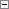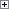An array is a data structure that contains several variables of the same type. The variables are treated as a single collection. Although you can already create arrays in C/AL, you can now use arrays from the .NET Framework. Choosing whether to use a C/AL array or a .NET Framework array depends on how you intend to use the data.

For this scenario Use this array type

Your C/AL code uses .NET Framework interoperability extensively, and you must share the array among many methods.

.NET Framework array

Your C/AL code mostly uses basic C/AL types and does not interoperate with the .NET Framework.

C/AL array

To use a .NET Framework array in C/AL, you must use the System.Array class in the .NET Framework class library, which is the class from which all arrays inherit. When interfacing with an array in C/AL, you must use the following methods of the System.Array class to create and access elements in the array.

Method Description

CreateInstance

Creates a new array instance that is passed to a method or property.

SetValue

Sets a value in an array element.

GetValue

Gets a value from an array element

Many .NET Framework classes implement these methods. Therefore, they are available for you to use in C/AL code. If you are writing your own .NET Framework code and want to use it in Microsoft Dynamics NAV, then you must implement these methods yourself.

In C/AL, some .NET Framework data types, such as strings, integers, and decimals, are automatically converted to C/AL types. If a .NET Framework array contains these data types, then you create an assembly that contains methods that return the information about the data types and then reference the assembly in the C/AL code. This concept is illustrated in the following examples.

Example: Using Native C/AL Data Types in a .NET Framework Array

The following example shows how you can use a .NET Framework array for an integer data type.

1. In Visual Studio, create a C# class library project called NavInteropHelper that contains a method that returns the information about the integer type as follows:
C#Copy Code
namespace Microsoft.Dynamics.Nav.NavInteropHelper;
{
public class WrapInt32
{
public static Type GetTypeOfInt32()
{
return typeof(Int32);
}
}
}

2. Build the project, and then copy the assembly to the Add-ins folder of the Microsoft Dynamics NAV Windows client installation folder. By default, the path of the installation folder is C:\Program Files\Microsoft Dynamics NAV\71RoleTailored Client or C:\Program Files (x86)\Microsoft Dynamics NAV\71RoleTailored Client.
3. In the development environment, open a Microsoft Dynamics NAV object, open the C/AL code, and then create the following C/AL variables.
Variable name DataType SubType

varDotNet

DotNet

'NavInteropHelper, Version=1.0.0.0, Culture=neutral, PublicKeyToken=null'.Microsoft.Dynamics.Nav.NavInteropHelper.WrapInt32

varArray

DotNet

'mscorlib, Version=2.0.0.0, Culture=neutral, PublicKeyToken=b77a5c561934e089'.System.Array

arraySize

Integer

i

Integer

alData

Integer

4. On a trigger of the Microsoft Dynamics NAV object, add the following C/AL code to create, fill, and implement the array.Copy Code
// Sets the number of elements in the .NET Framework array.
arraySize := 10;
// Creates an instance of the .NET Framework array that contains the Int32 type.
varArray := varArray.CreateInstance(varDotNet.GetTypeOfInt32(), arraySize);
// Clears the object instance because it is no longer used.
clear(varDotNet);
// Sets the data in the array.
FOR i := 0 TO (arraySize -1) DO
varArray.SetValue(i+100,i);
// Gets the array data and validates the content. The object that is returned by
// GetValue is automatically converted into a C/AL integer in the assignment.
FOR i := 0 TO (arraySize -1) DO
BEGIN
alData := varArray.GetValue(i);
if alData <> (i+100) then
error('Invalid array data in element %1, expected %2, actual %3', i, i+100, alData);
END;

Example: Using User-Defined Classes in a .NET Framework Array

This example shows how you can use a .NET Framework array that includes user-defined classes.

1. In Visual Studio, create a C# class library project called NavInteropHelper that contains methods that return the information about the types as follows.
C#Copy Code
namespace Microsoft.Dynamics.Nav.NavInteropHelper;
{
public class WrapInt32
{
public int Data { get; set; }
public WrapInt32(Int32 value)
{
Data = value;
}
}
}

2. Build the project, and then copy the assembly to the Add-ins folder of the Microsoft Dynamics NAV Windows client installation folder. By default, the path of the installation folder is C:\Program Files\Microsoft Dynamics NAV\71RoleTailored Client or C:\Program Files (x86)\Microsoft Dynamics NAV\71RoleTailored Client.
3. In the development environment, open a Microsoft Dynamics NAV object, open the C/AL code, and then create the following C/AL variables.
Variable name DataType SubType

varDotNet

DotNet

'NavInteropHelper, Version=1.0.0.0, Culture=neutral, PublicKeyToken=null'.Microsoft.Dynamics.Nav.NavInteropHelper.WrapInt32

varArray

DotNet

'mscorlib, Version=2.0.0.0, Culture=neutral, PublicKeyToken=b77a5c561934e089'.System.Array

arraySize

Integer

i

Integer

alData

Integer

4. On a trigger of the Microsoft Dynamics NAV object, add the following C/AL code to create, fill, and implement the array.Copy Code
// Sets the number of elements in the .NET Framework array.
arraySize := 10;
// Creates an instance of the .NET Framework object so the type information can be retrieved.
varDotNet := varDotNet.WrapInt32(1);
// Creates an instance of the .NET Framework array that contains the user-defined type.
varArray := varArray.CreateInstance(varDotNet.GetType(), arraySize);
// Clears the object instance because it is no longer used.
clear(varDotNet);
// Sets data in the array.
FOR i := 0 TO (arraySize -1) DO
BEGIN
varDotNet := varDotNet.WrapInt32(i+100);
varArray.SetValue(varDotNet,i);
END;
// Gets array data and validates content.
FOR i := 0 TO (arraySize -1) DO
BEGIN
varDotNet := varArray.GetValue(i);
// Gets the integer value from the .NET Framework object using the Data property.
alData := varDotNEt.Data;
if alData <> (i+100) then
error('Invalid array data in element %1, expected %2, actual %3', i, i+100, alData);
END;